#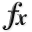N-D Test Functions Y¶

class go_benchmark.YaoLiu04(dimensions=2)

Yao-Liu 4 test objective function.

This class defines the Yao-Liu function 4 global optimization problem. This is a multimodal minimization problem defined as follows:Here,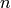represents the number of dimensions andfor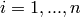.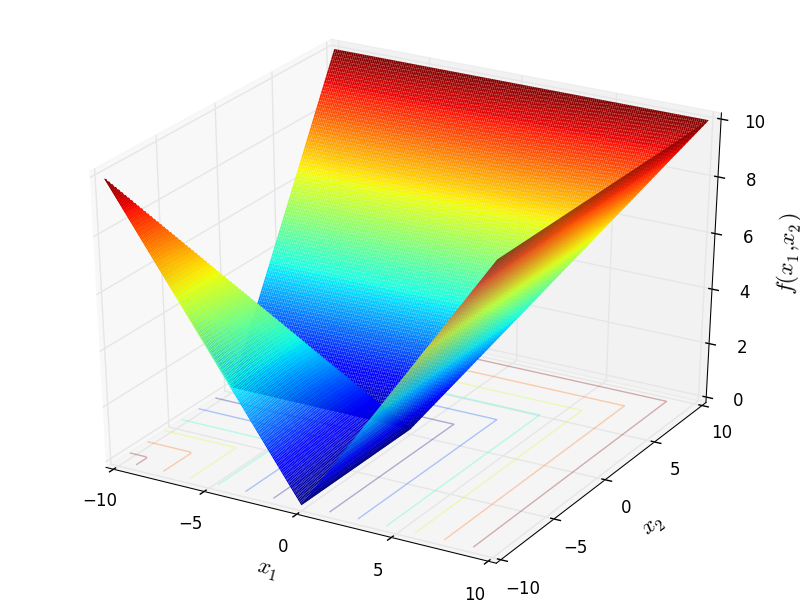Two-dimensional Yao-Liu 4 function

Global optimum: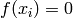for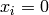forclass go_benchmark.YaoLiu09(dimensions=2)

Yao-Liu 9 test objective function.

This class defines the Yao-Liu function 9 global optimization problem. This is a multimodal minimization problem defined as follows: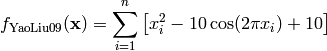Here,represents the number of dimensions and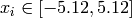for.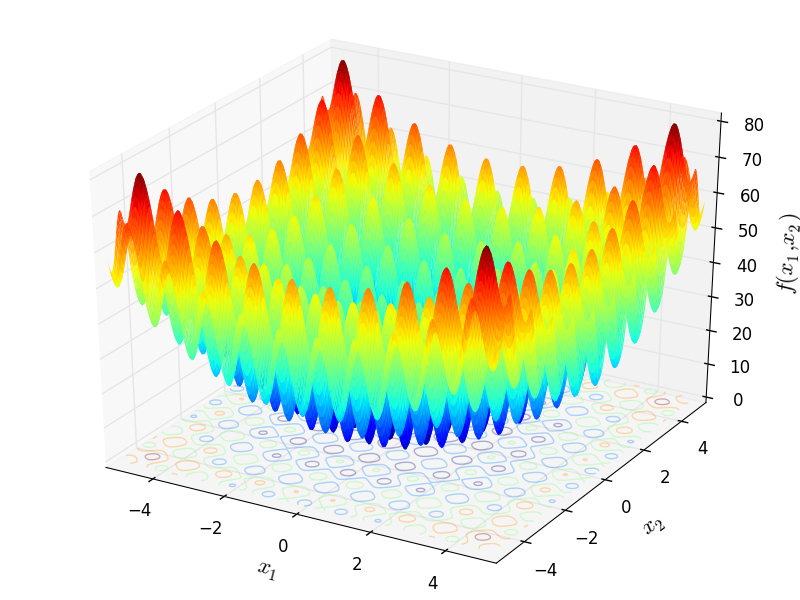Two-dimensional Yao-Liu 9 function

Global optimum:forfor#### Previous topic

N-D Test Functions X

#### Next topic

N-D Test Functions Z